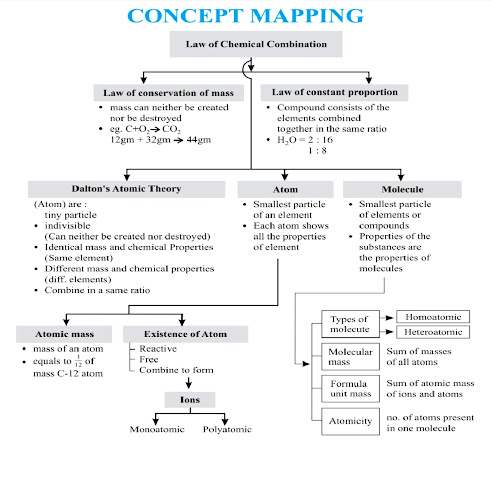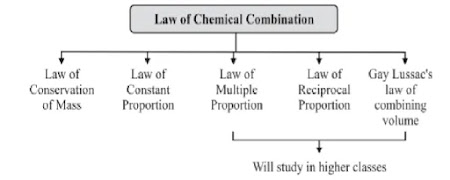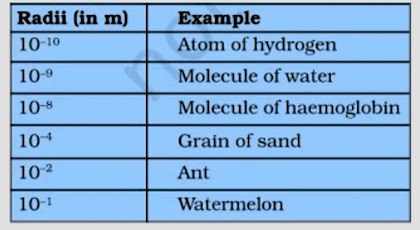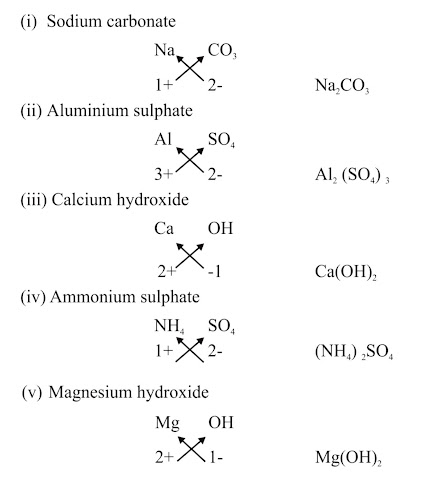9th Class Science Notes in English chapter 3 Atoms and Molecules

CBSE Revision Notes for CBSE Class 09 Science Atoms and Molecules Atoms and Molecules: Particle nature, basic units : Atoms and molecules. Law of constant proportions. Atomic and molecular masses for class 09 Science. It includes all main concepts of the chapter in CBSE class 09 Science.

Class 9th Science chapter 3 Atoms and Molecules Notes in English.

📚 Chapter - 3 📚
🔹Atoms and Molecules🔹✔ TOPICS IN THIS CHAPTER

🔹 Introduction

🔹 Laws of Chemical Combination

▶ Law of Conservation of Mass

▶ Law of Constant Proportions

🔹 Dalton's Atomic Theory

🔹 Atoms

▶ Modern day symbols of Elements

▶ Atomic Mass

▶ Atomic Mass Unit

▶ Atom existence

🔹 Molecules

🔹 Atomicity

🔹 Chemical Formulae

▶ Characteristics of chemical formulae

▶ Rules for writing chemical formulae

🔹 Molecular Mass

▶ Formula Unit Mass

🔹 Ions

🔹 Molar Mass

Mole Concept

Important Formulae

✔ Introduction:-

🔹  Around 500 B.C., Indian philosopher Maharishi Kanad, postulated the theory if we go on dividing matter (padarth), we will obtain smallest particle beyond which further division can't be possible which is known as 'parmanu'.

🔹  Ancient Greek philosophers  Democritus and Leucippus called these particles atoms.

🔹  Antoine L. Lavoisier said the foundation of chemical sciences by establishing two important laws of chemical combination.

✔ Laws of Chemical Combination:-

🔹 This law established after the experiments by Lavoisier and Joseph L. Proust.

🔹 The process of combination of two or more elements to form new compounds is governed by certain laws called laws of chemical combination. These are:1. Law of conservation of mass.

2. Law of constant proportions.

1. Law of Conservation of Mass (by Lavoisier in 1744):-

🔹 This law states that Mass can neither be created nor destroyed in a chemical reaction.

"During a chemical reaction, the total mass of reactants will be equal to the total mass of the products".

▶ Example:-   A (Reactant) + B (Reactant) AB  (Product)

mass of A + mass of B mass of AB

2. Law of constant proportions (by Proust in 1797):-

🔹This law states that in a chemical substance the elements are always present in definite proportions by mass.

▶ For Example:-      18 gm of H2O = 2 gm of hydrogen + 16 gm of oxygen

🔹mass of hydrogen/mass of oxygen = 2/16 = 1/8

(The ratio of hydrogen and oxygen in pure water is always 1: 8 by weight.)

- This law is also called law of definite proportions or law of constant proportions.

✔ Dalton’s Atomic Theory :-

🔹According to Dalton’s atomic theory, all matter, whether an element, a compound or a mixture is composed of small particles called atoms.

Six Postulates of Dalton's atomic theory:-

🔹 (I) All matter is made of very tiny particles called atoms.

🔹 (II) Atoms are indivisible particles, which cannot be created or destroyed in a chemical reaction.

🔹 (III) Atoms of a given element are identical in mass and chemical properties. (Law of conservation of mass)

🔹 (IV) Atoms of different elements have different masses and chemical properties.

🔹 (V) Atoms combine in the ratio of small whole numbers to form compounds. (Law of constant proportion)

🔹 (VI) The relative number and kinds of atoms are constant in a given compound.

✔ Atoms :-

🔹According to modern atomic theory, an atom is the smallest particle of an element which takes part in chemical reaction.

🔹Atoms are very small and hence can't be seen even through very powerful microscope.

🔹Atomic radius is measured in nanometres = 10⁻⁹ m▶ Modern day symbols of Elements :-

🔹Dalton was the first scientist to use the symbols for elements.

🔹Berzilius suggested that the symbols of elements should be made from one or two letters of the name of the element.Symbols of elements as proposed by Dalton

🔹The name copper was taken from Cyprus, a place from where it was found for first time.

🔹Now, IUPAC (International Union of Pure and Applied Chemistry) approves names of elements.

🔹The first letter of a symbol is always written as a capital letter (uppercase) and the second letter as a small letter (lowercase). For example: hydrogen (H), aluminium (Al), cobalt (co).

🔹Some other symbols have been taken from the names of elements in LatinGerman or Greek

▶ For Example:- Fe from its Latin name ferrumsodium is Na from natriumpotassium is K from kalium.Atomic Mass :-

🔹The mass of an atom of an element in called its atomic mass.

🔹In 1961, IUPAC have accepted 'atomic mass unit(u) to express atomic and molecular mass of elements and compounds.

▶ Atomic Mass Unit :-

🔹The atomic mass unit is defined as the quality of mass equal to 1/2 of mass of an atom of carbon- 12.

1 amu or u = 1/2 x Mass of an atom of C¹²
1 u = 1.66 x 10⁻²⁷ kg▶ Atomic Existance :-

🔹Atoms of most of the elements are very reactive and does not exist in free state.

🔹Only the atoms of noble gases (such as He, Ne, Ar. Kr. Xe and Rn) are chemically unreactive and can exist in the free state as single atom.

🔹Atoms of all other elements combine together to form molecules or ions.✔ Molecule :-

🔹A molecule is a group of two or more atoms which are chemically bonded with each other.

🔹A molecule is the smallest particle of matter (except element) which is capable of an independent existence and show all properties of that substance.

E.g.  'H₂O' is the smallest particle of water which shows all the properties of water.

🔹A molecule may have atom of same or different elements, depending upon this,

▶ Molecule can be categorized into two categories :-

🔹Homoatomic molecules (containing atom of same element)

▶ Example:-  H₂ , O₂ , O₃ , S₈ , P₄  etc.

🔹Heteroatomic molecules or compounds (containing atoms of different elements)

▶ Example:-  H₂O , CO₂ , NaCl , CaCO₃ etc.✔ Atomicity :-

🔹The number of atoms present in one molecule of an element is called its atomicity.✔ Chemical formulae :-

🔹It is the symbolic representation of the composition of a compound.

▶ Characteristics of chemical formulae :-

🔹The valencies or charges on ion must balance.

🔹When a compound is formed of metal and non-metal, symbol of metal comes first. É.g., CaO,         NaCl, CuO.

🔹When polyatomic ions are used, the ions are enclosed in brackets before writing the number to show the ratio. E.g., Ca(OH)₂ ,(NH₄)₂SO₄

▶ Rule for writing chemical formulae :-

Rule-1

🔹 (i) We first write symbols of elements which form compound.
🔹 (ii) Below the symbolo feach element, we should write the irvalency.
🔹 (iii) Now cross the valencies of combining atoms.
🔹 (iv) with first atom, we write the valeney of second atom (asa subscript).
🔹 (v) with second atom, we write the valency of first atom (subscript).

Rule-2

🔹 When the veleney is one, subscript is not written.

Rule-3

🔹 When there are multiple number of polyatomicion, bracket must be used to separate the  Polyatomicion from subscript.✔ Molecular Mass :-

🔹It is the sum of atomic masses of all the atoms in a molecule of that substance.

E.g. Molecular mass of H.O = 2 x Atomic mass of Hydrogen + 1 x Atomic mass of Oxygen

So, Molecular mass of H,O=2 x1 +1x 16= 18 u

▶ Formula Unit Mass :-

🔹It is the sum of atomic mass of ions and atoms present in formula for a compound

E.g.   In NaCl

Na = 23 a.m.u.
CI = 35.5 a,m.u.

So,Formula unit mass = 1 x 23 + 1x 35,5 = 58.5 u

✔ Ions :-

🔹An ion may be defined as an atom or group of atoms having positive or negative charge.

🔹Some positively charged ions: Na⁺, K⁺, Ca²⁺, Al³⁺

🔹Some negatively charged ions: Cr⁻ (chloride ion), s²⁻ (sulphide ion), OH⁻ (hydroxide ion), so₄²⁻, (sulphate ion)

🔹 We can classify ions in two types:-

▶ (1) Simple Ions

🔹 Mg²⁺ (Magnesium ion)
🔹 Na⁺ (Sodium ion)
🔹 cr⁻ (Chloride ion)
🔹 AI³⁺ (Aluminium ion)

▶ (ii) Compound Ions

🔹 NH⁴⁺ (Ammonium ion)
🔹 co₃²⁻ (Carbonate ion)
🔹 SO₄²⁻ (Sulphate ion)
🔹 OH⁻ (Hydroxide ion)

▶ Chemical Formulae of lonic Compounds (Polyatomic) :-✔ Molar Mass :-

🔹 The molar mass of a substance is the mass of I mole of that substance. It is equal to the 6.022 x 10" atoms of that element/substance.

▶ Example :-

🔹 (a) Atomic mass of hydrogen (H) is 1 u. Its molar mass is I g/mol.
🔹 (b) Atomic mass of nitrogen is 14 u. So, molar mass of nitrogen (N) is 14 g/mol.
🔹 (c) Molar mass of S₈ Mass of S x 8 = 32 x 8 = 256 g/mol
🔹 (d) Molar mass of HCI = Mass of H+ Mass of CI = 1 = 35.5 = 36.5 g/mol

▶ Mole concept :-

🔹A group of 6.022 x 10²³ Particles (atoms, molecules or ions) ofa substance is called a mole of that substance.

I mole of atoms         = 6.022 x 10²³ atoms
I mole of molecules = 6,022 x 10²³ molecules

▶ Example :-

I mole of oxygen = 6.022 x 10²³ oxygen atoms

6.022 x 10²³ is Avogadro Number (L).

🔹1 mole of atoms of an element has a mass equal to gram atomic mass of the element.

✔ Important Formulae :-